Question

# 30) At constant pressure and temperature, which statement is true? A) All reactions for which k...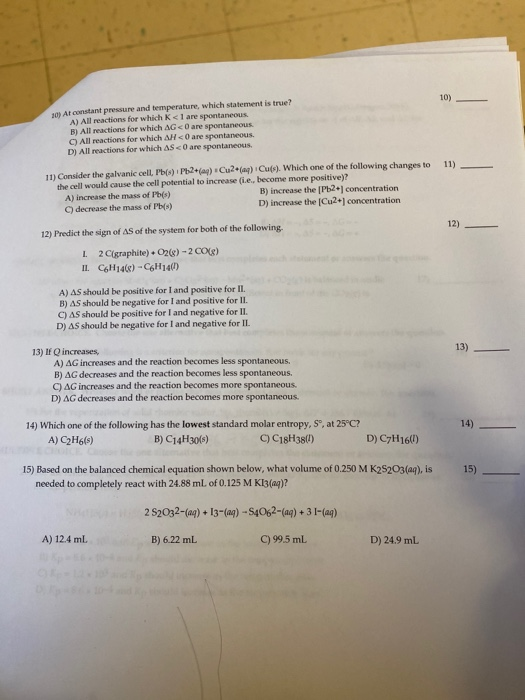30) At constant pressure and temperature, which statement is true? A) All reactions for which k < 1 are spontaneous B) All reactions for which AG are spontaneous C) All reactions for which AH<0 are spontaneous. D) All reactions for which AS <0 are spontaneous 11) 11) Consider the galvanic cell, Pb(s) IP20) Cu2+(a) Cu(s). Which one of the following changes to the cell would cause the cell potential to increase (ie, become more positive)? A) increase the mass of Pb(s) B) increase the [Pb2+ concentration decrease the mass of Pb(s) D) increase the Cu2+ concentration 12) 12) Predict the sign of AS of the system for both of the following 1 2 Cigraphite). O2(g) - 2018) II. C6H14() - CH1460 A) AS should be positive for I and positive for II. B) AS should be negative for I and positive for II. C) AS should be positive for I and negative for II. D) AS should be negative for I and negative for IL 13) If increases, A) AG increases and the reaction becomes less spontaneous. B) AG decreases and the reaction becomes less spontaneous. C) AG increases and the reaction becomes more spontaneous D) AG decreases and the reaction becomes more spontaneous 14) Which one of the following has the lowest standard molar entropy, S, at 25°C? A) C2H6(s) B) C14H30(s) C18H386) D) C7H16(1) 15) Based on the balanced chemical equation shown below, what volume of 0.250 MK2S2O3(aq), is needed to completely react with 24.88 mL of 0.125 M KI3(g)? 2S2O32- (aq) + 13- (aq) - S4062-(aq) +31-(aq) A) 12.4 mL B ) 6.22 mL C) 99.5 mL D) 24.9 mL

10)

K alone can’t decide whether the cell is spontaneous or not.

ΔG less than 0, implies the reaction is spontaneous.

ΔH and ΔS together can predict spontaneousity of the reaction.

Alone they cant.

11)

E = Eo - (0.0592/n) log {[Pb2+]/[Cu2+]}

To make E larger, we should decrease Pb2+ and increase Cu2+

Only 1 question at a time please

#### Earn Coins

Coins can be redeemed for fabulous gifts.

Similar Homework Help Questions
• ### A) AS should be positive for I and positive for II. B) AS should be negative...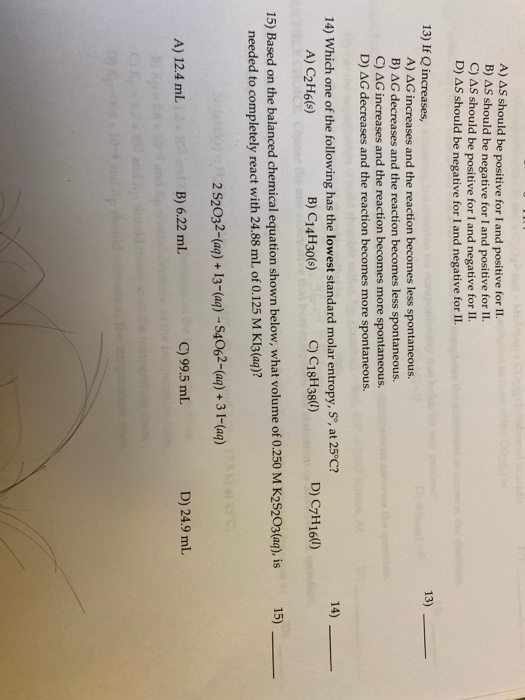A) AS should be positive for I and positive for II. B) AS should be negative for I and positive for II. C) AS should be positive for I and negative for II. D) AS should be negative for I and negative for II. 13) 13) If Q increases, A) AG increases and the reaction becomes less spontaneous. B) AG decreases and the reaction becomes less spontaneous. C) AG increases and the reaction becomes more spontaneous D) AG decreases and...

• ### Solve For a process at constant temperature and pressure, if DeltaG is positive, the process is,if...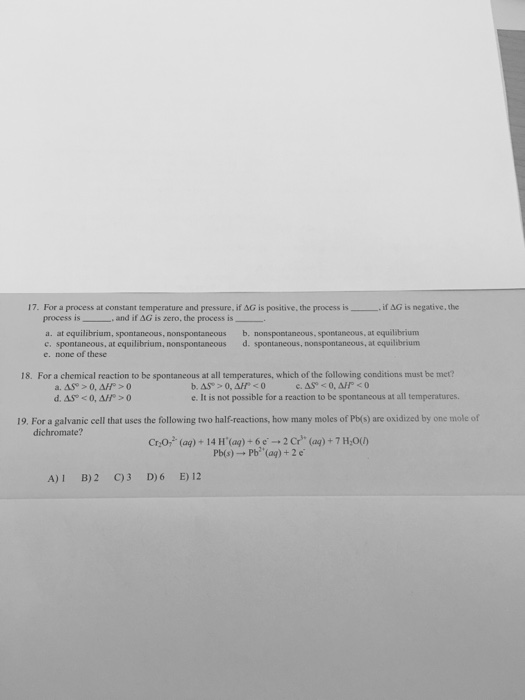Solve For a process at constant temperature and pressure, if DeltaG is positive, the process is,if DeltaG is negative, the process is, and if DeltaG is zero, the process is. a. at equilibrium, spontaneous, nonspontaneous b. nonspontaneous, spontaneous, at equilibrium c. spontaneous, at equilibrium, nonspontaneous d. spontaneous, nonspontaneous, at equilibrium e. none of these 18. For a chemical reaction to be spontaneous at all temperatures, which of the following conditions must be met? a. DeltaSdegree > 0, DeltaHdegree > 0...

• ### QUESTIONS At constant pressure and temperature, which statement is true? A. All reactions for which AH<0...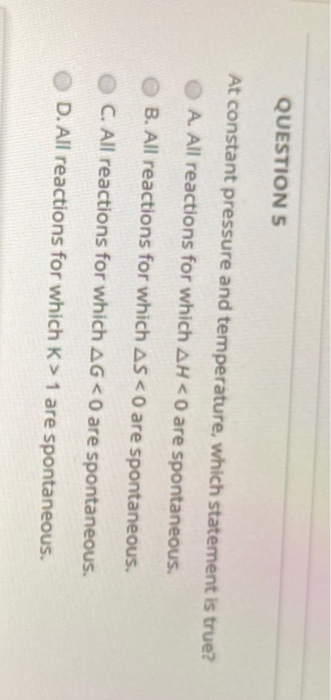QUESTIONS At constant pressure and temperature, which statement is true? A. All reactions for which AH<0 are spontaneous B. All reactions for which ASKO are spontaneous. C. All reactions for which AG<0 are spontaneous. D. All reactions for which K> 1 are spontaneous.

• ### 12) Predict the sign of AS of the system for both of the following. I. 2...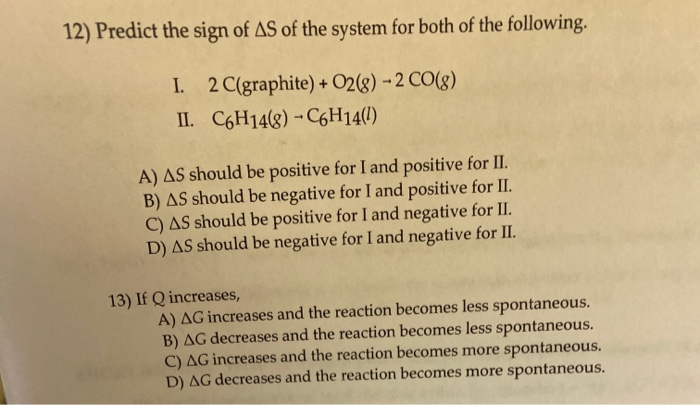12) Predict the sign of AS of the system for both of the following. I. 2 C(graphite) + O2(8) - 2 CO(8) II. C6H14(8) - C6H14(1) A) AS should be positive for I and positive for II. B) AS should be negative for I and positive for II. C) AS should be positive for I and negative for II. D) AS should be negative for I and negative for II. 13) If Q increases, A) AG increases and the reaction...

• ### please answer 24 and 25 Use this table to answer the question that follows. Thermodynamic Quantities...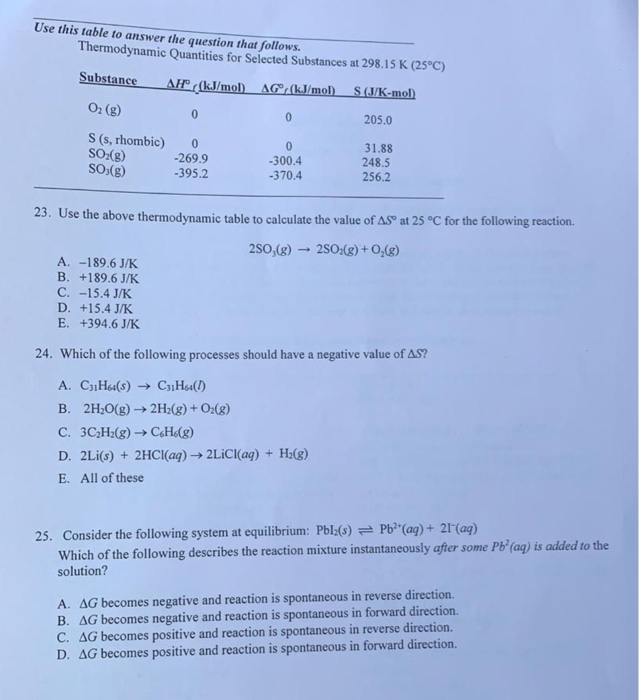please answer 24 and 25 Use this table to answer the question that follows. Thermodynamic Quantities for Selected Substances at 298.15 K (25°C) Substance AF(kJ/mol AG (kJ/mol). S(J/K-mol) O2 (g) 205.0 S (s, rhombic) SO2(g) SO (8) 0 -269.9 -395.2 -300.4 -370.4 31.88 248.5 256.2 23. Use the above thermodynamic table to calculate the value of ASO at 25 °C for the following reaction. 250,(8) - 2502(g) + O2(g) A. -189.6J/K B. +189.6 J/K C. -15.4 J/K D. +15.4 J/K...

• ### Which of the following would be true for a reaction that has a positive AS and...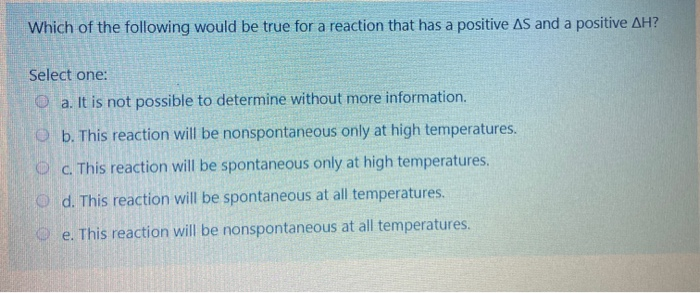Which of the following would be true for a reaction that has a positive AS and a positive AH? Select one: a. It is not possible to determine without more information. b. This reaction will be nonspontaneous only at high temperatures. c. This reaction will be spontaneous only at high temperatures. d. This reaction will be spontaneous at all temperatures. e. This reaction will be nonspontaneous at all temperatures. You prepared a galvanic cell using Pb(s) | Pb+2(aq) || Hg'...

• ### pls do all Page 12 of 14 36. (15 points) A spontaneous galvanic cell consists of one half-cell that e ontains Agia) and rea ad one half-cell that contains Cucs) and Cu2+(ag). The standard reductio...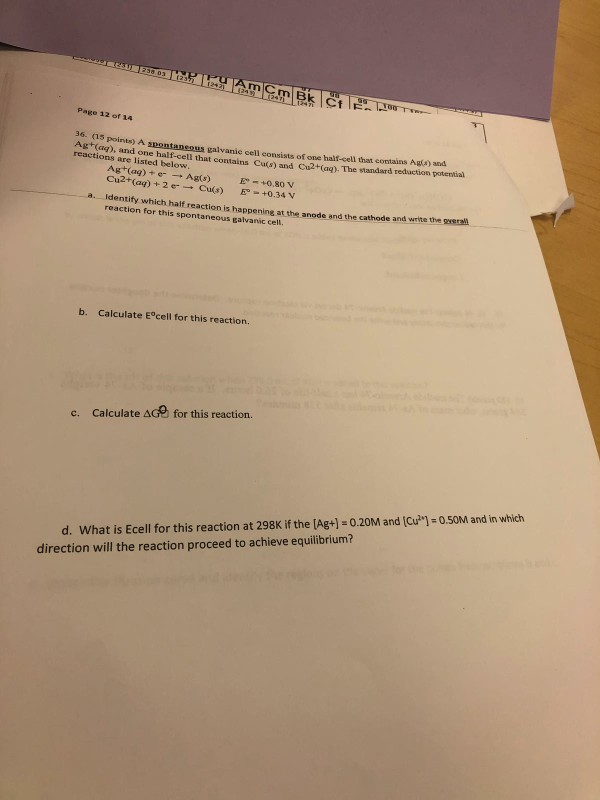pls do all Page 12 of 14 36. (15 points) A spontaneous galvanic cell consists of one half-cell that e ontains Agia) and rea ad one half-cell that contains Cucs) and Cu2+(ag). The standard reduction reactions are listed below Cu2+(aq) + 2 e-→ Cu(s) +0.34 V Identify which half reaction is happening at the anode and the cathode reaction for this spontaneous galvanic cell. a. and write the gwerall Calculate E°cell for this reaction. b. for this reaction. Calculate Δ...

• ### Amphiphilic molecules, such as phospholipids spontaneously form micelles/vesicles in water because: a) The entropy of the...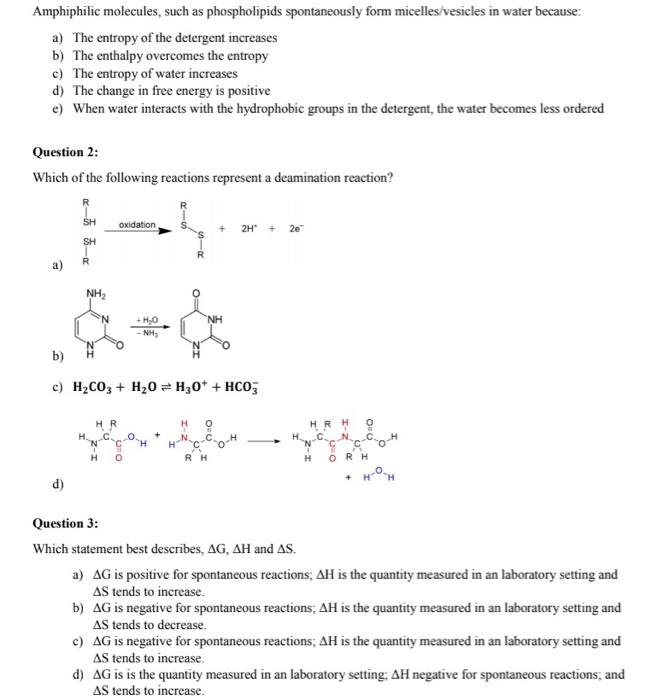Amphiphilic molecules, such as phospholipids spontaneously form micelles/vesicles in water because: a) The entropy of the detergent increases b) The enthalpy overcomes the entropy c) The entropy of water increases d) The change in free energy is positive e) When water interacts with the hydrophobic groups in the detergent, the water becomes less ordered Question 2: Which of the following reactions represent a deamination reaction? S + 2H + 2e c) H2CO3 + H20 = H30+ + HCO3 но нR...

• ### Please answer all parts to this question! parts a through e! This is all one question...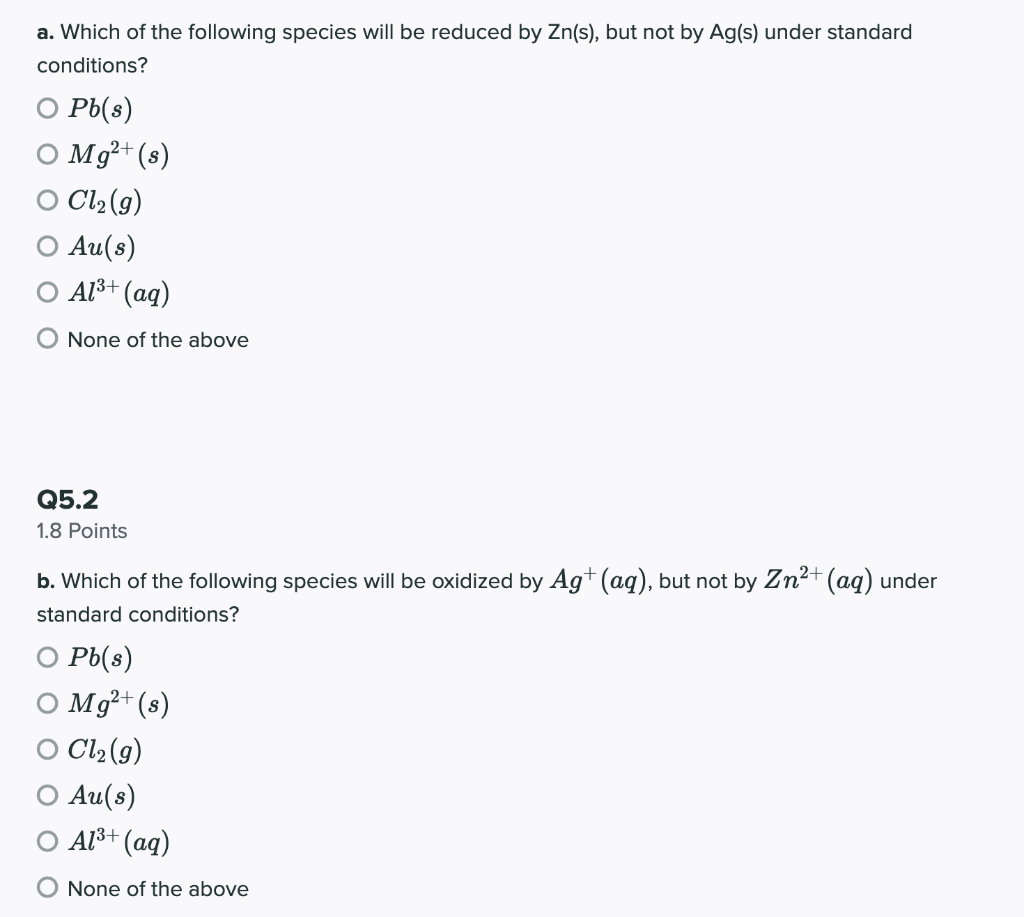Please answer all parts to this question! parts a through e! This is all one question so I could not post them separately, please also check your answers because the last person got it wrong! Thank you! Will give thumbs up. a. Which of the following species will be reduced by Zn(s), but not by Ag(s) under standard conditions? O Pb(s) O Mg2+(s) O Cl2(g) O Au(s) O A13+ (aq) O None of the above Q5.2 1.8 Points b. Which...

• ### Enter electrons as e Use smallest possible integer coefficients for ALL reactions, If a box is...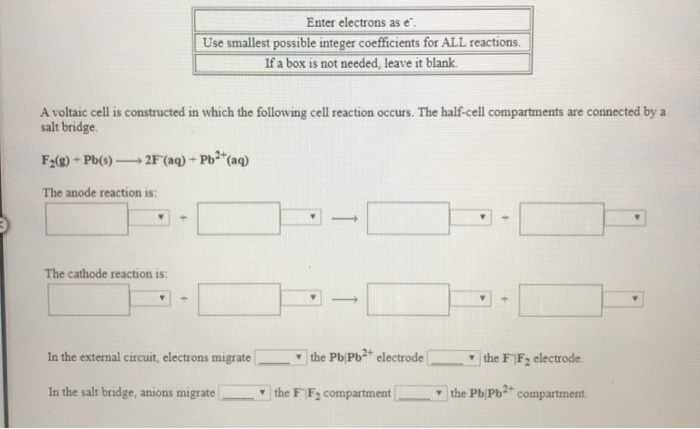Enter electrons as e Use smallest possible integer coefficients for ALL reactions, If a box is not needed, leave it blank. A voltaic cell is constructed in which the following cell reaction occurs. The half-cell compartments are connected by a salt bridge. F2(g) + Pb(s) — 2F (aq) + Pb2+(aq) The anode reaction is: The cathode reaction is: In the external circuit, electrons migrate the Pb/Pb2+ electrode the FF, electrode In the salt bridge, anions migrate the FF2 compartment the...